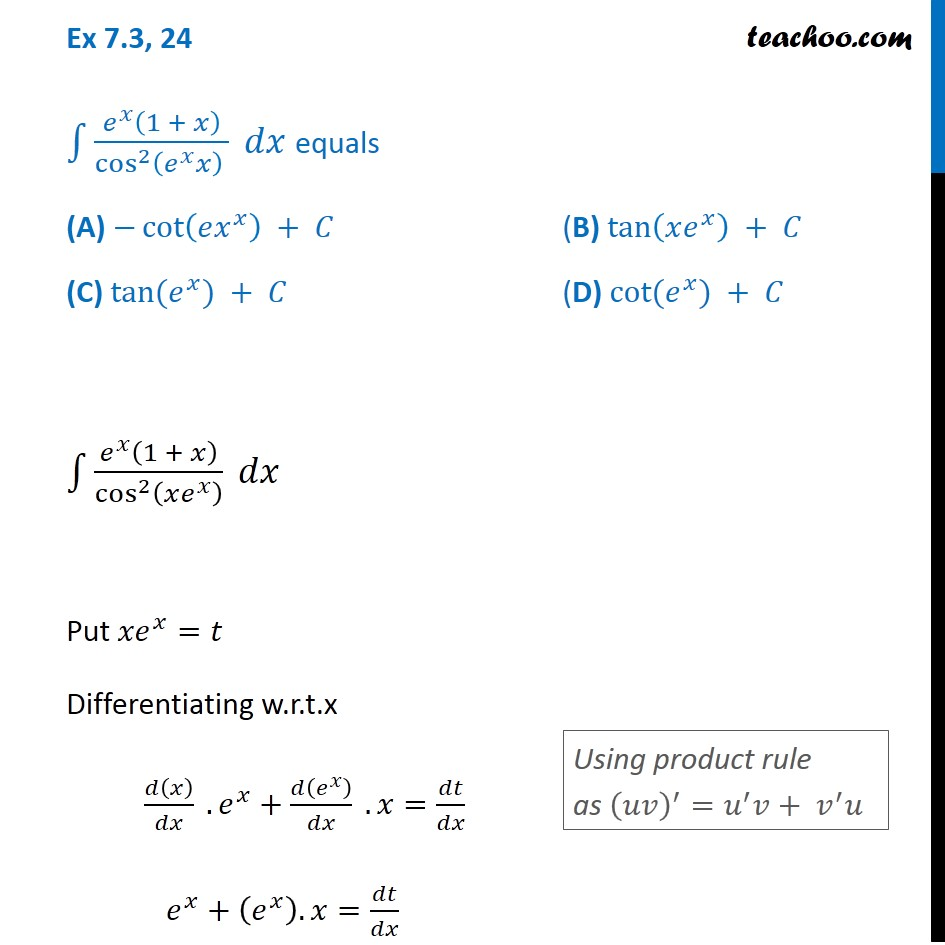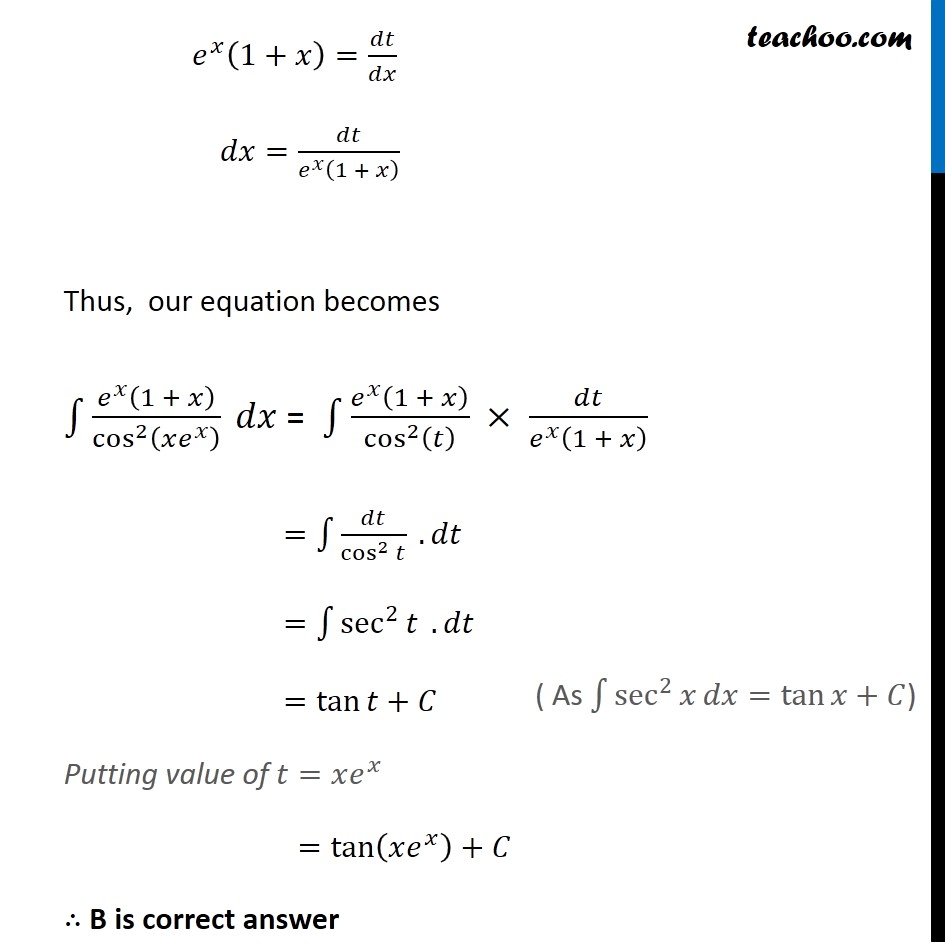Ex 7.3

Chapter 7 Class 12 Integrals
Serial order wiseLearn in your speed, with individual attention - Teachoo Maths 1-on-1 Class

### Transcript

Ex 7.3, 24 ∫1▒(𝑒^𝑥 (1 + 𝑥))/(cos^2⁡(𝑒^𝑥 𝑥) ) 𝑑𝑥 equals (A) −cot⁡(𝑒𝑥^𝑥 ) + 𝐶 (B) tan⁡(𝑥𝑒^𝑥 ) + 𝐶 (C) tan⁡(𝑒^𝑥) + 𝐶 (D) cot⁡(𝑒^𝑥) + 𝐶 ∫1▒(𝑒^𝑥 (1 + 𝑥))/cos^2⁡(𝑥𝑒^𝑥 ) 𝑑𝑥 Put 〖𝑥𝑒〗^𝑥=𝑡 Differentiating w.r.t.x 𝑑(𝑥)/𝑑𝑥 . 𝑒^𝑥+𝑑(𝑒^𝑥 )/𝑑𝑥 . 𝑥=𝑑𝑡/𝑑𝑥 𝑒^𝑥+(𝑒^𝑥 ). 𝑥=𝑑𝑡/𝑑𝑥 Using product rule as (𝑢𝑣)^′=𝑢^′ 𝑣+ 𝑣^′ 𝑢 𝑒^𝑥 (1+𝑥)=𝑑𝑡/𝑑𝑥 𝑑𝑥=𝑑𝑡/(𝑒^𝑥 (1 + 𝑥) ) Thus, our equation becomes ∫1▒(𝑒^𝑥 (1 + 𝑥))/cos^2⁡(𝑥𝑒^𝑥 ) 𝑑𝑥 = ∫1▒(𝑒^𝑥 (1 + 𝑥))/cos^2⁡(𝑡) × 𝑑𝑡/(𝑒^𝑥 (1 + 𝑥) ) =∫1▒𝑑𝑡/cos^2⁡𝑡 . 𝑑𝑡 =∫1▒sec^2⁡𝑡 . 𝑑𝑡 =tan⁡𝑡+𝐶 Putting value of 𝑡=𝑥𝑒^𝑥 =tan⁡(𝑥𝑒^𝑥 )+𝐶 ∴ B is correct answer ( As ∫1▒sec^2⁡𝑥 𝑑𝑥=tan⁡𝑥+𝐶)